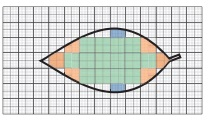Home | | Maths 6th Std | Area of Irregular Shapes

# Area of Irregular Shapes

The area of the shapes like triangle, square etc., are found by standard formulae. But we can find the approximate area of shapes like leaves as follows.

Area of Irregular Shapes

The area of the shapes like triangle, square etc., are found by standard formulae. But we can find the approximate area of shapes like leaves as follows.Place a leaf on a graph sheet and trace its boundary. Now observe the squares of size 1 cm × 1 cm inside of this boundary. We get complete squares (Green), partial but bigger than half squares (Orange) and half squares (Blue). The smaller than half squares which have negligible area are omitted.Now the approximate area of the leaf

= Number of full squares + Number of more than half squares + 1/2 × Number of half squares

= (14 + 6 + ½ × 2) sq. cm

= 21 sq. cm

Note

You will learn to find the actual area of irregular shapes like leaves in your higher classes.

Try these

Find the approximate area of the following figures:Tags : Term 3 Chapter 3 | 6th Maths , 6th Maths : Term 3 Unit 3 : Perimeter and Area
Study Material, Lecturing Notes, Assignment, Reference, Wiki description explanation, brief detail
6th Maths : Term 3 Unit 3 : Perimeter and Area : Area of Irregular Shapes | Term 3 Chapter 3 | 6th Maths